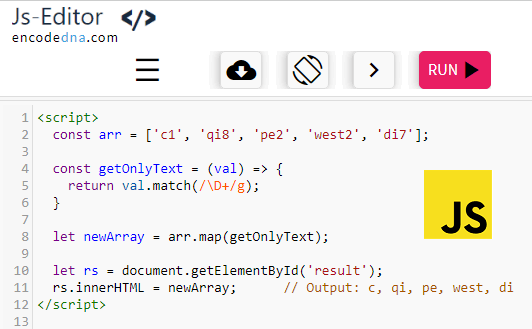﻿ How to get only letters from Array values in JavaScript

# How to get only letters from Array values in JavaScript

Let us assume I have an array that has string values like this … ['c1', 'pe2', 'qi8']. How do you get or extract the letters (like c, pe and qi) from the array? You can do this using the map() function in JavaScript with Regex. Let us see how.

imageThe Code
```<body>
<p id='result'></p>
</body>
<script>
const arr = ['c1', 'qi8', 'pe2', 'west2', 'di7'];

const getOnlyText = (val) => {
return val.match(/\D+/g);
}

let newArray = arr.map(getOnlyText);

let rs = document.getElementById('result');
rs.innerHTML = newArray;
</script>
</html>```

The map() function creates a new array from another array. It internally iterates each element in the array.

Along with the map, I am also using the match() function. The match returns an array with the matches. But, what should it return? I want the function to return only text values.

I have provided Regex /\D+/g as an argument to the match() function.

The capital D gets all the text values (the alphabets). However, D will return the first alphabet only. Therefore, I have used D+.

The g in regex, does a global search.

Now do this, and see what it returns.

return val.match(/\D/);
OR
return val.match(/\d/);

The match() function in the above example will throw an error if the array has numbers or numeric values. Suppose the array has an numeric value 34, along with string values...

`const arr = ['c1', 'qi8', 'pe2', 'west2', 'di7', 34];`

All except one is a number (34). You can filter out the number from the array and then use match().

```const getOnlyText = (val) => {
if (typeof(val) !== 'number') {
return val.match(/\D+/g);
}
}```

That's it.